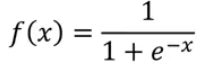## Pages

Machine Learning Quiz (134 Objective Questions) Start ML Quiz

Deep Learning Quiz (205 Objective Questions) Start DL Quiz

## Saturday, 15 June 2019

### Difference between Sigmoid and Softmax function in deep learning

Softmax function can be understood as a generalized version of a sigmoid function or an extension of a sigmoid function. Softmax function is usually used in the output layers of neural networks.

Following are some of the differences between Sigmoid and Softmax function:

1. The sigmoid function is used for the two-class (binary) classification problem, whereas the softmax function is used for the multi-class classification problem.

2. Sum of all softmax units are supposed to be 1. In sigmoid, it’s not really necessary. Sigmoid just makes output between 0 to 1. The softmax enforces that the sum of the probabilities of all the output classes are equal to one, so in order to increase the probability of a particular class, softmax must correspondingly decrease the probability of at least one of the other classes.

When you use a softmax, basically you get a probability of each class (join distribution and a multinomial likelihood) whose sum is bound to be one. In case, you use sigmoid for multi class classification, it’d be like a marginal distribution and a Bernoulli likelihood.

3. Formula for Sigmoid and Softmax

Sigmoid function:Softmax function:

Let me illustrate the point 2 with an example here. Lets say, we have 6 inputs:

[1,2,3,4,5,6]

If we pass these inputs through the sigmoid function, we will get following output:

[0.5, 0.73, 0.88, 0.95, 0.98, 0.99]

Sum of the above output units is 5.03 which is greater than 1.

But in case of softmax, the sum of output units is always 1. Lets see how? Pass the same input to softmax function, and we get following output:

[0.001, 0.009, 0.03, 0.06, 0.1, 0.8] which sums up to 1.

4. Sigmoid is usually used as an activation function in hidden layers (but we use ReLU nowadays) while Softmax is used in output layers.

A general rule of thumb is to use ReLU as an activation function in hidden layers and softmax in output layer in a neural networks. For more information on activation functions, please visit my this post.